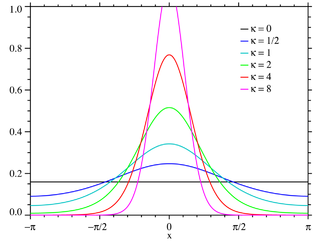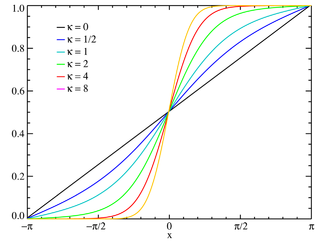# von Mises distribution

﻿
von Mises distribution
parameters: Probability density functionThe support is chosen to be [-π,π] with μ=0 Cumulative distribution functionThe support is chosen to be [-π,π] with μ=0 μ real κ > 0$x\in$ any interval of length 2π$\frac{e^{\kappa\cos(x-\mu)}}{2\pi I_0(\kappa)}$ (not analytic - see text) μ μ μ var(x) = 1 − I1(κ) / I0(κ) (circular)$-\kappa\frac{I_1(\kappa)}{I_0(\kappa)}+\ln[2\pi I_0(\kappa)]$ (differential)$\frac{I_{|n|}(\kappa)}{I_0(\kappa)}e^{i n \mu}$

In probability theory and directional statistics, the von Mises distribution (also known as the circular normal distribution or Tikhonov distribution) is a continuous probability distribution on the circle. It is a close approximation to the wrapped normal distribution, which is the circular analogue of the normal distribution. A freely diffusing angle θ on a circle is a wrapped normally distributed random variable with an unwrapped variance that grows linearly in time. On the other hand, the von Mises distribution is the stationary distribution of a drift and diffusion process on the circle in a harmonic potential, i.e. with a preferred orientation . The von Mises distribution is the maximum entropy distribution for a given expectation value of z = eiθ. The von Mises distribution is a special case of the von Mises-Fisher distribution on the N-dimensional sphere.

The von Mises probability density function for the angle x is given by:$f(x|\mu,\kappa)=\frac{e^{\kappa\cos(x-\mu)}}{2\pi I_0(\kappa)}$

where I0(x) is the modified Bessel function of order 0.

The parameters μ and 1/κ are analogous to μ and σ2 (the mean and variance) in the normal distribution:

• μ is a measure of location (the distribution is clustered around μ), and
• κ is a measure of concentration (a reciprocal measure of dispersion, so 1/κ is analogous to σ2).
• If κ is zero, the distribution is uniform, and for small κ, it is close to uniform.
• If κ is large, the distribution becomes very concentrated about the angle μ with κ being a measure of the concentration. In fact, as κ increases, the distribution approaches a normal distribution in x  with mean μ and variance 1/κ.

The probability density can be expressed as a series of Bessel functions (see Abramowitz and Stegun §9.6.34)$f(x|\mu,\kappa)=\,$$\frac{1}{2\pi}\left(1\!+\!\frac{2}{I_0(\kappa)} \sum_{j=1}^\infty I_j(\kappa)\cos[j(x\!-\!\mu)]\right)$

where Ij(x) is the modified Bessel function of order j. The cumulative distribution function is not analytic and is best found by integrating the above series. The indefinite integral of the probability density is:$\Phi(x|\mu,\kappa)=\int f(t|\mu,\kappa)\,dt=$$\frac{1}{2\pi}\left(x\!+\!\frac{2}{I_0(\kappa)} \sum_{j=1}^\infty I_j(\kappa)\frac{\sin[j(x\!-\!\mu)]}{j}\right).$

The cumulative distribution function will be a function of the lower limit of integration x0:$F(x|\mu,\kappa)=\Phi(x|\mu,\kappa)-\Phi(x_0|\mu,\kappa).\,$

## Moments

The moments of the von Mises distribution are usually calculated as the moments of z = eix rather than the angle x itself. These moments are referred to as "circular moments". The variance calculated from these moments is referred to as the "circular variance". The one exception to this is that the "mean" usually refers to the argument of the circular mean, rather than the circular mean itself.

The nth raw moment of z is:$m_n=\langle z^n\rangle=\int_\Gamma z^n\,f(x|\mu,\kappa)\,dx$$= \frac{I_{|n|}(\kappa)}{I_0(\kappa)}e^{i n \mu}$

where the integral is over any interval Γ of length 2π. In calculating the above integral, we use the fact that zn = cos(nx) + i sin(nx) and the Bessel function identity (See Abramowitz and Stegun §9.6.19):$I_n(\kappa)=\frac{1}{\pi}\int_0^\pi e^{\kappa\cos(x)}\cos(nx)\,dx.$

The mean of z  is then just$m_1= \frac{I_1(\kappa)}{I_0(\kappa)}e^{i\mu}$

and the "mean" value of x is then taken to be the argument μ. This is the "average" direction of the angular random variables. The variance of z, or the circular variance of x is:$\textrm{var}(x)= 1-E[\cos(x-\mu)] = 1-\frac{I_1(\kappa)}{I_0(\kappa)}.$

## Limiting behavior

In the limit of large κ the distribution becomes a normal distribution$\lim_{\kappa\rightarrow\infty} f(x|\mu,\kappa)=\frac{\exp\left[\dfrac{-(x-\mu)^2}{2\sigma^2}\right]}{\sigma\sqrt{2\pi}}$

where σ2 = 1/κ. In the limit of small κ it becomes a uniform distribution:$\lim_{\kappa\rightarrow 0}f(x|\mu,\kappa)=\mathrm{U}(x)$

where the interval for the uniform distribution U(x) is the chosen interval of length 2π.

## Estimation of parameters

A series of N measurements$z_n=e^{i\theta_n}$ drawn from a von Mises distribution may be used to estimate certain parameters of the distribution. (Borradaile, 2003) The average of the series$\overline{z}$ is defined as$\overline{z}=\frac{1}{N}\sum_{n=1}^N z_n$

and its expectation value will be just the first moment:$\langle\overline{z}\rangle=\frac{I_1(\kappa)}{I_0(\kappa)}e^{i\mu}.$

In other words,$\overline{z}$ is an unbiased estimator of the first moment. If we assume that the mean μ lies in the interval [ − π,π), then Arg$(\overline{z})$ will be a (biased) estimator of the mean μ.

Viewing the zn as a set of vectors in the complex plane, the$\bar{R}^ 2$ statistic is the square of the length of the averaged vector:$\bar{R}^ 2=\overline{z}\,\overline{z^*}=\left(\frac{1}{N}\sum_{n=1}^N \cos\theta_n\right)^2+\left(\frac{1}{N}\sum_{n=1}^N \sin\theta_n\right)^2$

and its expectation value is:$\langle \bar{R}^2\rangle=\frac{1}{N}+\frac{N-1}{N}\,\frac{I_1(\kappa)^2}{I_0(\kappa)^2}.$

In other words, the statistic$R_e^2=\frac{N}{N-1}\left(\bar{R}^2-\frac{1}{N}\right)$

will be an unbiased estimator of$\frac{I_1(\kappa)^2}{I_0(\kappa)^2}\,$ and solving the equation$R_e=\frac{I_1(\kappa)}{I_0(\kappa)}\,$ for$\kappa\,$ will yield a (biased) estimator of$\kappa\,$. In analogy to the linear case, the solution to the equation$\bar{R}=\frac{I_1(\kappa)}{I_0(\kappa)}\,$ will yield the maximum likelihood estimate of$\kappa\,$ and both will be equal in the limit of large N.

## Distribution of the mean

The distribution of the sample mean$\overline{z} = \bar{R}e^{i\overline{\theta}}$ for the von Mises distribution is given by:$P(\bar{R},\bar{\theta})\,d\bar{R}\,d\bar{\theta}=\frac{1}{ (2\pi I_0(k))^N}\int_\Gamma \prod_{n=1}^N \left( e^{\kappa\cos(\theta_n-\mu)} d\theta_n\right) = \frac{e^{\kappa N\bar{R}\cos(\bar{\theta}-\mu)}}{I_0(\kappa)^N}\left(\frac{1}{(2\pi)^N}\int_\Gamma \prod_{n=1}^N d\theta_n\right)$

where N is the number of measurements and$\Gamma\,$ consists of intervals of in the variables, subject to the constraint that$\bar{R}$ and$\bar{\theta}$ are constant, where$\bar{R}$ is the mean resultant:$\bar{R}^2=|\bar{z}|^2= \left(\frac{1}{N}\sum_{n=1}^N \cos(\theta_n) \right)^2 + \left(\frac{1}{N}\sum_{n=1}^N \sin(\theta_n) \right)^2$

and$\overline{\theta}$ is the mean angle:$\overline{\theta}=\mathrm{Arg}(\overline{z}). \,$

Note that product term in parentheses is just the distribution of the mean for a circular uniform distribution.

## Entropy

The information entropy of the Von Mises distribution is defined as:$H = -\int_\Gamma f(\theta;\mu,\kappa)\,\ln(f(\theta;\mu,\kappa))\,d\theta\,$

where Γ is any interval of length . The logarithm of the density of the Von Mises distribution is straightforward:$\ln(f(\theta;\mu,\kappa))=-\ln(2\pi I_0(\kappa))+ \kappa \cos(\theta)\,$

The characteristic function representation for the Von Mises distribution is:$f(\theta;\mu,\kappa) =\frac{1}{2\pi}\left(1+2\sum_{n=1}^\infty\phi_n\cos(n\theta)\right)$

where ϕn = I | n | (κ) / I0(κ). Substituting these expressions into the entropy integral, exchanging the order of integration and summation, and using the orthogonality of the cosines, the entropy may be written:$H = \ln(2\pi I_0(\kappa))-\kappa\phi_1 = \ln(2\pi I_0(\kappa))-\kappa\frac{I_1(\kappa)}{I_0(\kappa)}$

For κ = 0, the Von Mises distribution becomes the circular uniform distribution and the entropy attains its maximum value of ln(2π).

Wikimedia Foundation. 2010.

### Look at other dictionaries:

• Von Mises distribution — Probability distribution name =von Mises type =density pdf The support is chosen to be [ π,π] with μ=0 cdf The support is chosen to be [ π,π] with μ=0 parameters =mu real kappa>0 support =xin any interval of length 2π pdf =frac{e^{kappacos(x… …   Wikipedia

• von Mises — may refer to: Ludwig von Mises Institute von Mises distribution, named after Richard von Mises von Mises yield criterion, named after Richard von Mises People Dr. Mises, pseudonym of Gustav Fechner Ludwig von Mises, economist of the Austrian… …   Wikipedia

• Von Mises — may refer to:* Ludwig von Mises, famous economist of the Austrian School * Richard von Mises, mathematician, younger brother of Ludwig von Mises * von Mises distribution * Ludwig von Mises Institute * von Mises yield criterion * Dr. Mises,… …   Wikipedia

• Von Mises–Fisher distribution — The von Mises–Fisher distribution is a probability distribution on the (p 1) dimensional sphere in mathbb{R}^{p}. If p=2the distribution reduces to the von Mises distribution on the circle. The distributionbelongs to the field of directional… …   Wikipedia

• Cramér–von Mises criterion — In statistics the Cramér–von Mises criterion is a criterion used for judging the goodness of fit of a cumulative distribution function F * compared to a given empirical distribution function Fn, or for comparing two empirical distributions. It is …   Wikipedia

• Cramér-von-Mises criterion — In statistics the Cramér von Mises criterion is a form of minimum distance estimation used for judging the goodness of fit of a probability distribution F^* compared to a given distribution F is given by:n W^2 = n int { infty}^{infty} [F(x)… …   Wikipedia

• Ludwig von Mises — Ludwig Heinrich Edler von Mises Austrian School Born 29 September 1881(1881 09 29) Lemberg …   Wikipedia

• Hilda Geiringer von Mises — (publizierte als Hilda Geiringer oder Hilda Pollaczek Geiringer; verheiratete von Mises; * 28. September 1893 in Wien; † 22. März 1973 in Santa Barbara (Kalifornien)) war eine österreichisch US amerikanische Mathematikerin, die sich mit… …   Deutsch Wikipedia

• Cramér-von-Mises-Test — Der Cramér von Mises Test ist ein statistischer Test, mit dem untersucht werden kann, ob die Häufigkeitsverteilung der Daten einer Stichprobe von einer vorgegebenen hypothetischen Wahrscheinlichkeitsverteilung abweicht (Ein Stichproben Fall),… …   Deutsch Wikipedia

• Maximum entropy probability distribution — In statistics and information theory, a maximum entropy probability distribution is a probability distribution whose entropy is at least as great as that of all other members of a specified class of distributions. According to the principle of… …   Wikipedia